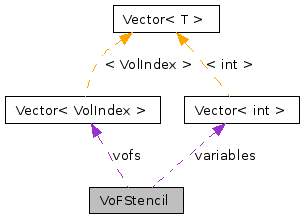# VoFStencil Class Reference

`#include <Stencils.H>`

Collaboration diagram for VoFStencil:[legend]

## Detailed Description

VoF-centered stencil.

This stencil is a fundamental tool for building eb applications by making the ability to cache stencil information. This object consists of a vector of VoFs and a vector of weights.

## Public Member Functions

VoFStencil ()
~VoFStencil ()
void clear ()
void add (const VolIndex &vof, const Real &weight, int ivar=0)
int size () const
const VolIndexvof (int i) const
const Realweight (int i) const
Realweight (int i)
const int & variable (int i) const
int & variable (int i)
VoFStenciloperator+= (const VoFStencil &a_vofsten)
void operator*= (const Real &scaling)
VoFStenciloperator= (const VoFStencil &a_vofsten)
VoFStencil (const VoFStencil &a_vofstenin)
void setAllVariables (int a_var)

## Protected Attributes

Vector< VolIndexvofs
the VoFs
Vector< Realweights
the weights
Vector< int > variables

## Constructor & Destructor Documentation

 VoFStencil::VoFStencil ( )

default constructor. makes empty vectors.

 VoFStencil::~VoFStencil ( )

 VoFStencil::VoFStencil ( const VoFStencil & a_vofstenin )

## Member Function Documentation

 void VoFStencil::clear ( )

 void VoFStencil::add ( const VolIndex & vof, const Real & weight, int ivar = `0` )

add a VoF to the Stencil, with it's associated weight it is required that all VoFs are in the same EBIndexSpace If the vof is already in the stencil, add the weights.

 int VoFStencil::size ( ) const` [inline]`

number of VoFs in the Stencil

inlines

References weights.

 const VolIndex & VoFStencil::vof ( int i ) const` [inline]`

access a VoF

References vofs.

 const Real & VoFStencil::weight ( int i ) const` [inline]`

access a weight

References weights.

 Real & VoFStencil::weight ( int i ) ` [inline]`

access a weight

References weights.

 const int& VoFStencil::variable ( int i ) const

 int& VoFStencil::variable ( int i )

 VoFStencil& VoFStencil::operator+= ( const VoFStencil & a_vofsten )

add all faces and weights of inputs to this. If a vof is already in the stencil, add the weights. only addition is well-defined here as far as arithmatic operations are concerned.

 void VoFStencil::operator*= ( const Real & scaling )

 VoFStencil& VoFStencil::operator= ( const VoFStencil & a_vofsten )

 void VoFStencil::setAllVariables ( int a_var ) ` [inline]`

References Vector< T >::size(), variables, and vofs.

## Member Data Documentation

 Vector VoFStencil::vofs` [protected]`

the VoFs

Referenced by setAllVariables(), and vof().

 Vector VoFStencil::weights` [protected]`

the weights

Referenced by size(), and weight().

 Vector VoFStencil::variables` [protected]`

Referenced by setAllVariables().

The documentation for this class was generated from the following file:

Generated on Tue Apr 14 14:24:06 2009 for Chombo + EB by1.5.5